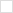TWiki>P1076 Web>Vhdl2019CollectedRequirements>RealMatrixPackage (2020-02-17, JimLewis)

# Real Matrix Package

## Proposal Information

• Who Updates: DavidBishop, JimLewis, ...
• Date Proposed: 2013-11-13
• Date Last Updated: 2013-11-13
• Priority:
• Complexity:
• Focus: Testbench

## Summary

Implement real matrix package. Package is required for 1076.1.

## Proposed Changes - David's Summary of the discussion

1) In the packages I reference a matrix with A(row,col). Matlab references with A(col, row). The change would involve reversing the index of every funciton. Pro: Make it more like matlab and less like C Con: Would take several days to make the change.

2) Ability to flatten a matrix into a vector.

3) Remove the polyval, tril, triu, blkdiag functions Pro: These are standard Matlab function. Con: There are typically only used it Matlab demonstrations...

4) better documentaiton for "buildmatrix" function.

5) "randn" function Creates a random matrix with a gausian destribution.

6) create versions of the "zeros" and "ones" and other matrix initalizing commands which take a matrix as an argument (just to get the size). Thus you could say: a <= zeros (5,5); a <= zeros (a);

7) Use "positive" ranges instead of "natural" ranges. This would make the matrix package work more like Matlab. However an address of "(0,0)" would be illegal. The VHDL-2008 predefined types for index_vector and real_vector include zero, so this would be a disconnect.

8) Check ranges to make sure that it is always 1 to x and not "downto" x.

9) "**" operator This operator is recursive is the power is greater than 2. Should someting greather than 2 create an error?

10) The function polyval takes two real_vectors arguments and returns real_vector. This definition is, in my opinion, questionable because in all my life I have never had a need to determine a polynomial value for a vector argument, the argument was always a scalar. This most common use case of a polynomial evaluated for a scalar is made rather complicated: var := polyval (coeff, (0=>x))(0); I recommend to either change the definition of polyval or to add an overloaded version with a scalar argument and scalar return type. Also, change the names of the formal arguments to indicate which is the coefficients.

11) The algorithm used for polyval is numerically poor, both from the perspective of accuracy and performance. According to several books on numerical algebra I consulted Horner's method is much preferable here.

12) There a strict distinction between the mathematical concepts of row vectors and column vectors. A row vector is represented as a VHDL vector, a column vector as a VHDL matrix with a single column. While you have defined transpose functions to convert between row and column vector I foresee overloading ambiguities requiring qualified expressions, which adds complexity. Another approach would be to use a convention: if the left argument of a function is a VHDL vector it's considered a row vector. If the right argument of a function is a VHDL vector it's considered a column vector. I believe this convention works for all the functions taking a real_vector as argument, including mrdivide and mldivide.

13) I propose to add "*"(l, r: real_vector) as an equivalent to dot()

14) Equivalent functions: should they be defined as separate declarations or as aliases? I haven't thought through all the implications of one approach or the other, but the package currently maintains separate definitions (e.g. "/", mrdivide)

15) Modify the "x, y" inputs to be "row, column"

1) Addressing the Matrix via A(row, col) works fine now.

2) To flatten a matrix you can use the "reshape" command (as documented in Matlab)

vec := reshape (mat, 1, mat'length(1)*mat'length(2))

works.

4) Documented this function.

6) Created "size_res" version of zeros, ones, eye, rand, and reshape functions.

8) Created a function to check the ranges. Should this be an error or a warning? The algorithms are designed so that they don't care.

## Suggested Changes

1) The matrix packages make use of none of the new expressive capabilities introduced by the 2008 revision of VHDL. There are currently 4 variants defined of the matrix packages: real, integer, complex, and fixed-point. Presumably, there are many similarities between the packages, especially in defining the standard matrix operations. Unfortunately, this method of defining closely related packages can lead to inconsistencies in the implementations. This is a good opportunity to demonstrate the usefullness of the new capabilities added to the VHDL language in the most recent standard revision.

The 2008 revision of VHDL introduced generic packages to the language. Generic packages allow the element type of a matrix to be specified as an interface type. The interface type should be packaged along with operations that are valid for the type. I would suggest the following definition of a matrix element package as a starting point:

```package matrix_element is
generic( type elem_t;
zero : elem_t;
unit : elem_t;
function "+"(x1,x2 : elem_t) return elem_t is <>;
function "*"(x1,x2 : elem_t) return elem_t is <>
);
type matrix_t is array(natural range <>, natural range <>) of elem_t;
end package matrix_element;

```

The matrix_element package then works like an algebraic concept. It can be associated with an interface package in a package that implements the matrix operations:

```package matrix_ops is
generic( package mx_pack is new work.matrix_element generic map(<>) );
use mx_pack.all;
function "+"(m1,m2 : matrix_t) return matrix_t;
...
end package matrix_ops;```

2) The operators of the matrix packages do not deal with ranges of incoming parameters flexibly. In particular, it seems that there is an assumption that all ranges will be TO, rather than DOWNTO. Consequently, elements are combined based on their indexed values, rather than their positions. The correct method for referencing elements of matrices and vectors is to declare aliases of parameters with canonical ranges, as is done in the IEEE numeric_bit package, say with the ADD_UNSIGNED function:

```function ADD_UNSIGNED(L, R : UNSIGNED; C: BIT) return UNSIGNED is
constant L_LEFT : INTEGER := L'left-1;
alias XL        : UNSIGNED(L_LEFT downto 0) is L;...

```

-- CliffordWalinsky - 2014-09-03